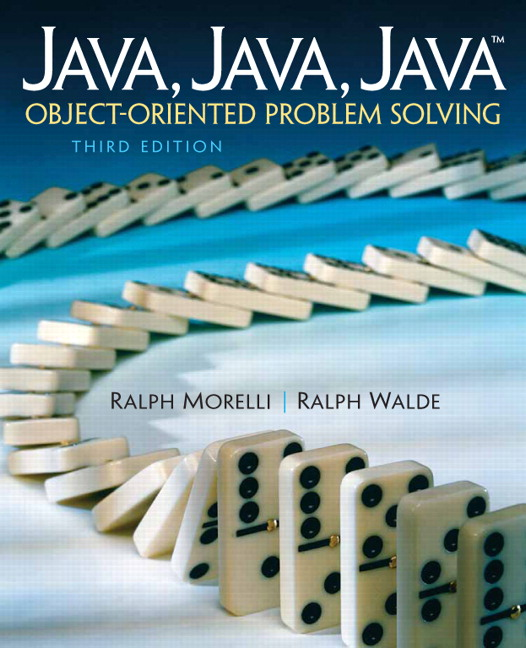# Java, Java, Java: Object-Oriented Problem Solving, 2022E

## Section5.1Introduction

This chapter has two primary goals. One is to elaborate on Java’s primitive data types, which were first introduced in Chapter 1.
We will cover boolean, integer, character, and real number data types, including the various operations that you can perform on these types. We will provide examples, including several modifications of the OneRowNim class, to show typical uses of the various data types.
Our second goal is to illustrate the idea that programming is a matter of choosing an appropriate way to represent a problem as well as choosing an appropriate sequence of actions to solve the problem. Programming is a form of problem solving that can be viewed as a two-part process: representation and action.
Representation means finding a way to look at the problem. This might involve seeing the problem as closely related to a known problem or seeing that parts of the problem can be broken up into smaller problems that you already know how to solve. In terms of programming problems, representation often means choosing the right kinds of objects and structures.
Action is the process of taking well-defined steps to solve a problem. Given a particular way of representing the problem, what steps must we take to arrive at its solution?
Choosing an appropriate representation is often the key to solving a problem. For example, consider this problem: Can a chess board, with its top-left and bottom-right squares removed, be completely tiled by dominoes that cover two squares at a time?
One way to solve this problem might be to represent the chess board and dominoes as shown in Figure 5.1.1. If we represent the board in this way, then the actions needed to arrive at a solution involve searching for a tiling that completely covers the board. In other words, we can try one way of placing the dominoes on the board. If that doesn’t work, we try another way. And so on. This process will be very time consuming, because there are millions of different ways of trying to tile the board.